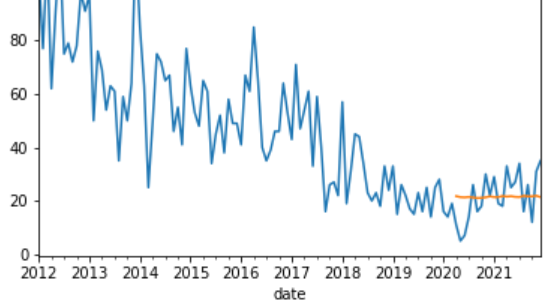# LSTM Prediction Flat Line but close shape

I created an LSTM but the prediction is always very close to a straight line. I normalised the train and test set separately (fit on train then transform the whole dataset).

``````train_size = int(len(B1_monthly_df) * 0.8)
B1_monthly_df_training = B1_monthly_df.copy()
train_data = B1_monthly_df_training.iloc[:train_size]

sc = MinMaxScaler()
#fit only on train data
scaler = sc.fit(train_data[train_data.columns])
#scale entire dataset
B1_monthly_df_training[B1_monthly_df_training.columns] = scaler.transform(B1_monthly_df_training[B1_monthly_df_training.columns])
``````

After I inverse transformed the predictions, this is what I got (blue is actual, orange is predicted)However when I isolated the actual and predicted and plotted them in their own scales, the shapes seem to be quite similar (and the mean is around the same scale [around 20 for both]), just that the scale of variation in predicted (around 0.8) is so much smaller than in train (around 30).

I also tested out using a more months for test (60% test, 40% train) to see if it was just because the last part is flattish, but I still got a very straight line.

What could be the cause of such an issue? Thanks!